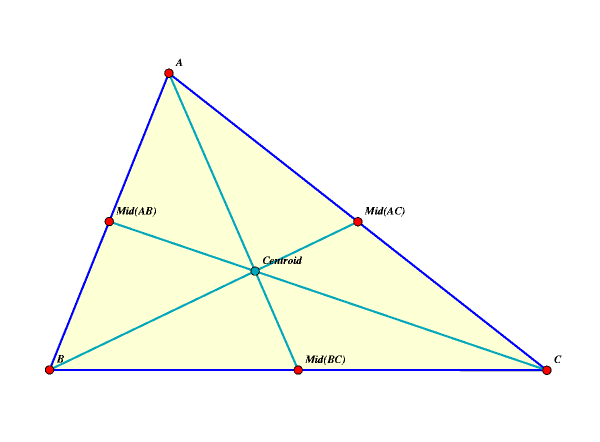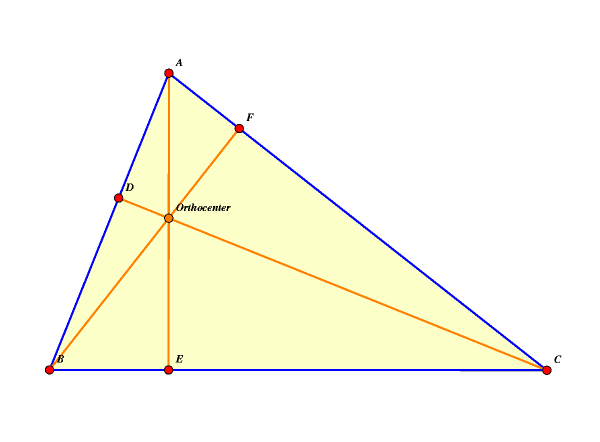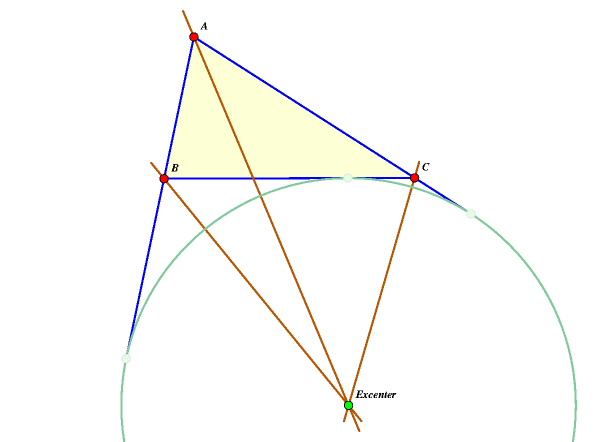# Various Triangle Centers

Below are several figures for various points related to triangles. These include the centroid, the circumcenter, the orthocenter, the incenter, the excenters, and the Euler line (which is a line, rather than a point-- can you trust anything I say?).

This page requires a java-enabled browser for correct functioning. The bright red points (A, B, and C) can be moved around with the mouse and the figure will adjust accordingly.

 The centroid of a triangle is the point at which the three medians meet. A median is the line between a vertex and the midpoint of the opposite side.The three perpendicular bisectors of the sides of a triangle meet at the circumcenter. The circumcenter is also the center of the circle passing through the three vertices, which circumscribes the triangle. This circle is sometimes called the circumcircle.
 The orthocenter is the point of intersection of the altitudes of the triangle, that is, the perpendicular lines between each vertex and the opposite side. When the orthocenter is combined with the three vertices, any one of the points is the orthocenter of the other three. This same property holds for the set of four points consisting of the incenter and the three excenters. Such points are said to form an orthocentric system. Any three points from an orthocentric system all have the same nine-point circle associated with them.The incenter of the triangle is the point at which the three bisectors of the interior angles of the triangle meet. This is also the center of the inscribed circle, also called the incircle of the triangle.
 An excenter is a point at which the line bisecting one interior angle meets the bisectors of the two exterior angles on the opposite side. This is the center of a circle, called an excircle which is tangent to one side of the triangle and the extensions of the other two sides. Naturally, every triangle has three excenters and three excircles.The Euler line of a triangle is the line that passes through the orthocenter, the circumcenter, and the centroid. It also contains the center of the Nine Point Circle.

Java images created using Cinderella by Scott Sutherland on March 12, 2004.